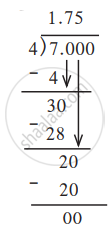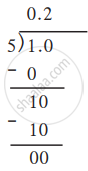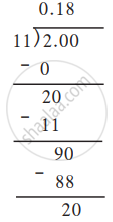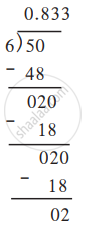# Decimal Representation of Rational Numbers

## Notes

### Decimal Representation of Rational Numbers:

1) Write the rational number 7/4 in decimal form.(1) 7 = 7.0 = 7.000 (Any number of zeros can be added after the fractional part.)

(2) 1 is the quotient and 3 the remainder after dividing 7 by 4. Now we place a decimal point after the integer 1. Writing the 0 from the dividend after the remainder 3, we divide 30 by 4. As the quotient, we get now is fractional, we write 7 after the decimal point. Again we bring down the next 0 from the dividend and complete the division.

2) Write 2 1/5 in decimal form.

We shall find the decimal form of 2 1/5 = 11/5 in three different ways.

Find the decimal form of 1/5.∴ 1/5 = 0.2

3) Write the number 2/11 in decimal form.∴ 2/11 = 0.1818.......

2/11 = 0.bar18

4) Work out the decimal form of 5/6.5/6 = 0.833...

∴ 5/6 = 0.8dot3

Here, a single digit or a group of digits occurs repeatedly on the right of the decimal point. This type of decimal form of a rational number is called the recurring decimal form.

If in a decimal fraction, a single-digit occurs repeatedly on the right of the decimal the point, we put a point above it as shown here. 5/6 = 0.8dot3 and if a group of digits occurs repeatedly, we show it with a horizontal line above the digits. Thus, 2/11 = 0.bar18.

If you would like to contribute notes or other learning material, please submit them using the button below.

### Shaalaa.com

Decimal Representation of Rational Numbers [00:06:17]
S
0%